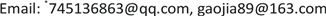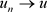﻿ 含Φ-Laplace算子的拟线性椭圆型方程的无穷多解 Infinitely Many Solutions for Quasilinear Elliptic Equations with Φ-Laplacian Operator

Pure Mathematics
Vol. 09  No. 10 ( 2019 ), Article ID: 33289 , 9 pages
10.12677/PM.2019.910138

Infinitely Many Solutions for Quasilinear Elliptic Equations with Φ-Laplacian Operator

Mingmin Wang*, Gao Jia

College of Science, University of Shanghai for Science and Technology, ShanghaiReceived: Nov. 14th, 2019; accepted: Nov. 27th, 2019; published: Dec. 4th, 2019ABSTRACT

By using fountain theorem, we studied a class of the Dirichlet boundary value problems of quasilinear elliptic equation with Φ-Laplacian operator. Without the Ambrosetti-Rabinowitz condition, we obtained infinitely many weak solutions.

Keywords:Quasilinear Elliptic Equation, Concave-Convex Nonlinearity, Fountain Theorem1. 引言

$\left\{\begin{array}{l}-{\Delta }_{\Phi }u=\lambda {u}^{\tau -1}+f\left(x,u\right),\text{}x\in \Omega ,\\ u=0,\text{}\text{ }\text{}x\in \partial \Omega ,\end{array}$ (1)

$\left\{\begin{array}{l}-\text{div}\left(a\left(|\nabla u|\right)\nabla u\right)=f\left(x,u\right),\text{}x\in \Omega ,\\ u=0,\text{}x\in \partial \Omega ,\end{array}$ (2)

$\left({\varphi }_{1}\right)$$t\to 0$ 时， $t\varphi \left(t\right)\to 0$，当 $t\to \infty$ 时， $t\varphi \left(t\right)\to \infty$

$\left({\varphi }_{2}\right)$ $t\varphi \left(t\right)$$\left(0,+\infty \right)$ 上严格增的；

$\left({\varphi }_{3}\right)$ $0<\mathcal{l}-1\le \frac{{\left(t\varphi \left(t\right)\right)}^{\prime }}{\varphi \left(t\right)}\le m-1<{\mathcal{l}}^{\ast }-1<ℕ$，其中 ${\mathcal{l}}^{\ast }=\frac{N\mathcal{l}}{N-\mathcal{l}}$$0<\tau <\mathcal{l}$

$\left({\varphi }_{4}\right)$ 存在常数 ${\mu }_{1}>0$，使得对任意的 $a\in \left[0,1\right]$$t>0$，有

$\stackrel{¯}{\Phi }\left(at\right)\le \stackrel{¯}{\Phi }\left(t\right)+{\mu }_{1}$

$\left({f}_{1}\right)$ f满足次临界增长条件，即对任意的 $\left(x,t\right)\in \Omega ×ℝ$，有

$|f\left(x,t\right)|\le {c}_{0}\left(1+{|t|}^{q-1}\right)$

$\left({f}_{2}\right)$ $\underset{|t|\to \infty }{\text{lim}}\frac{F\left(x,t\right)}{{|t|}^{m}}=+\infty$ 对几乎所有 $x\in \Omega$ 一致成立；

$\left({f}_{3}\right)$ 存在常数 ${\mu }_{2}>0$，使得对任意的 $a\in \left[0,1\right]$，有

$\stackrel{¯}{F}\left(x,at\right)\le \stackrel{¯}{F}\left(x,t\right)+{\mu }_{2}$$\forall \left(x,t\right)\in \Omega ×ℝ$

$\left({f}_{4}\right)$ $f\left(x,-t\right)=-f\left(x,t\right)$$\forall \left(x,t\right)\in \Omega ×ℝ$

${\int }_{\Omega }\Phi \left(|\nabla {u}_{k}|\right)\text{d}x-\frac{\lambda }{\tau }{\int }_{\Omega }{|{u}_{k}|}^{\tau }\text{d}x-{\int }_{\Omega }F\left(x,{u}_{k}\right)\text{d}x\to \infty$$k\to \infty$

2. 预备知识和基本引理

${L}_{\Phi }\left(\Omega \right)=\left\{u|u:\Omega \to ℝ是可测的,{\int }_{\Omega }\Phi \left(|u\left(x\right)|\right)\text{d}x<\infty \right\}$，在 ${L}_{\Phi }\left(\Omega \right)$ 上定义Luxemburg范数：

${‖u‖}_{\Phi }=\underset{k}{\mathrm{inf}}\left\{k>0|{\int }_{\Omega }\Phi \left(\frac{|u\left(x\right)|}{k}\right)\text{d}x\le 1\right\}$

${W}^{1,\Phi }\left(\Omega \right)=\left\{u|u\in {L}_{\Phi }\left(\Omega \right),{D}_{i}u\in {L}_{\Phi }\left(\Omega \right),i=1,\cdots ,n\right\}$，在 ${W}^{1,\Phi }\left(\Omega \right)$ 上定义范数：

${‖u‖}_{1,\Phi }={‖u‖}_{\Phi }+{‖\nabla u‖}_{\Phi }$

${W}_{0}^{1,\Phi }\left(\Omega \right)$${C}_{0}^{\infty }\left(\Omega \right)$${W}^{1,\Phi }\left(\Omega \right)$ 中的闭包。设 $\Phi$ 满足 ${\Delta }_{\text{2}}$ -条件，即 $\Phi \left(2t\right)\le K\Phi \left(t\right)$$\forall t\ge 0$，则 ${L}_{\Phi }\left(\Omega \right)$${W}^{1,\Phi }\left(\Omega \right)$ 是可分、自反的Banach空间(见文献  )。

${d}_{\Omega }=diam\left(\Omega \right)$，则对任意 $u\in {W}_{0}^{1,\Phi }\left(\Omega \right)$，有 ${\int }_{\Omega }\Phi \left(u\right)\text{d}x\le {\int }_{\Omega }\Phi \left(2{d}_{\Omega }|\nabla u|\right)\text{d}x$，那么 ${‖u‖}_{\Phi }\le 2{d}_{\Omega }{‖\nabla u‖}_{\Phi }$。因此，定义在 ${W}_{0}^{1,\Phi }\left(\Omega \right)$ 上的范数 $‖u‖:={‖\nabla u‖}_{\Phi }$${‖\cdot ‖}_{1,\Phi }$ 等价。

${\Phi }_{*}^{-1}={\int }_{0}^{t}\frac{{\Phi }^{-1}\left(s\right)}{{s}^{\frac{N+1}{N}}}\text{d}s$，且当 $t<0$ 时， ${\Phi }_{\ast }\left(t\right)={\Phi }_{\ast }\left(-t\right)$。如果对于所有的 $\nu >0$，都有 $\underset{t\to \infty }{\mathrm{lim}}\frac{\Upsilon \left(\nu t\right)}{{\Phi }_{\ast }\left(t\right)}=0$，则称函数 $\Upsilon$ 在无穷远处比 ${\Phi }_{\ast }$ 增长得更慢，记 $\Upsilon \ll {\Phi }_{\ast }$。如果 $\Upsilon \ll {\Phi }_{\ast }$，则。进一步，有。在条件 $\left({\varphi }_{1}\right)\text{-}\left({\varphi }_{3}\right)$ 下，有连续嵌入：$\mathcal{l}-2\le \frac{{\varphi }^{\prime }\left(t\right)t}{\varphi \left(t\right)}\le m-2$$\mathcal{l}\le \frac{\varphi \left(t\right){t}^{2}}{\Phi \left(t\right)}\le m$$t>0$

${\eta }_{1}\left(t\right)=\mathrm{min}\left\{{t}^{\mathcal{l}},{t}^{m}\right\}$${\eta }_{2}\left(t\right)=\mathrm{max}\left\{{t}^{\mathcal{l}},{t}^{m}\right\}$

${\eta }_{1}\left(t\right)\varphi \left(\rho \right)\le \varphi \left(\rho t\right)\le {\eta }_{2}\left(t\right)\varphi \left(\rho \right)$${\eta }_{\text{1}}\left({‖u‖}_{\Phi }\right)\le {\int }_{\Omega }\Phi \left(u\right)\text{d}x\le {\eta }_{\text{2}}\left({‖u‖}_{\Phi }\right)$

$X=\stackrel{¯}{\text{span}\left\{{e}_{j}:j=1,2,\cdots \right\}}$${X}^{\ast }=\stackrel{¯}{\text{span}\left\{{e}_{j}^{\ast }:j=1,2,\cdots \right\}}$

$〈{e}_{j},{e}_{j}^{\ast }〉=\left\{\begin{array}{l}1,\text{}i=j,\\ 0,\text{}i\ne j.\end{array}$

${X}_{j}=\text{span}\left\{{e}_{j}\right\}$，对任意的 $k\in ℕ$，定义 ${Y}_{k}=\underset{j=1}{\overset{k}{\oplus }}{X}_{j}$${Z}_{k}=\stackrel{¯}{\underset{j=k}{\overset{\infty }{\oplus }}{X}_{j}}$

i) ${a}_{k}:=\underset{u\in {Z}_{k},‖u‖={r}_{k}}{\mathrm{inf}}I\left(u\right)\to +\infty$$k\to \infty$

ii) ${b}_{k}:=\underset{u\in {Y}_{k},‖u‖={\rho }_{k}}{\mathrm{max}}I\left(u\right)\le 0$

iii) 对任意 $c>0$，I满足 ${\left(C\right)}_{c}$ 条件；

${I}_{\lambda }\left(u\right)={\int }_{\Omega }\Phi \left(|\nabla u|\right)\text{d}x-\frac{\lambda }{\tau }{\int }_{\Omega }{|u|}^{\tau }\text{d}x-{\int }_{\Omega }F\left(x,u\right)\text{d}x$(3)

${{I}^{\prime }}_{\lambda }\left(u\right)v={\int }_{\Omega }\varphi \left(|\nabla u|\right)\nabla u\nabla v\text{d}x-\lambda {\int }_{\Omega }{|u|}^{\tau -\text{2}}uv\text{d}x-{\int }_{\Omega }f\left(x,u\right)v\text{d}x$$\forall v\in {W}_{0}^{1,\Phi }\left(\Omega \right)$

3. 主要结果的证明

${I}_{\lambda }\left({u}_{n}\right)\to c$$n\to \infty$(4)

${‖{{I}^{\prime }}_{\lambda }\left({u}_{n}\right)‖}_{\ast }\left(1+‖{u}_{n}‖\right)\to 0$$n\to \infty$(5)

${I}_{\lambda }\left({u}_{n}\right)+o\left(1\right)=c$(6)

$〈{{I}^{\prime }}_{\lambda }\left({u}_{n}\right),v〉=o\left(1\right)$(7)

$\underset{n\to \infty }{\mathrm{lim}}‖{u}_{n}‖=\infty$，且 $‖{u}_{n}‖>1$$\forall n\in ℕ$

${w}_{n}:=\frac{{u}_{n}}{‖{u}_{n}‖}$$n=1,2,\cdots$，则 $\left\{{w}_{n}\right\}\subset {W}_{0}^{1,\Phi }\left(\Omega \right)$$‖{w}_{n}‖=1$$\forall n\in ℕ$，因此，存在子列(仍记为其本身)，

${w}_{n}⇀w$ (在 ${W}_{0}^{1,\Phi }\left(\Omega \right)$ 中)， (8)

${w}_{n}\to w$ (在 ${L}^{q}\left(\Omega \right)$ 中)， (9)

${w}_{n}\to w$ $a.e.\text{\hspace{0.17em}}x\in \Omega$(10)

$\frac{F\left(x,{u}_{n}\right)}{{‖{u}_{n}‖}^{m}}=\frac{F\left(x,{u}_{n}\right)}{{|{u}_{n}|}^{m}}{|{w}_{n}|}^{m}\to \infty$$n\to \infty$

$\frac{F\left(x,t\right)}{{|t|}^{m}}>1$(11)

$|F\left(x,t\right)|\le {c}_{1}$(12)

$c+o\left(1\right)={I}_{\lambda }\left({u}_{n}\right)={\int }_{\Omega }\Phi \left(|\nabla {u}_{n}|\right)\text{d}x-\frac{\lambda }{\tau }{\int }_{\Omega }{|{u}_{n}|}^{\tau }\text{d}x-{\int }_{\Omega }F\left(x,{u}_{n}\right)\text{d}x$

$\begin{array}{c}\underset{n\to \infty }{\underset{_}{\mathrm{lim}}}{\int }_{\Omega }\frac{F\left(x,{u}_{n}\right)}{{‖{u}_{n}‖}^{m}}\text{d}x=\frac{\underset{n\to \infty }{\underset{_}{\mathrm{lim}}}{\int }_{\Omega }\Phi \left(|\nabla {u}_{n}|\right)\text{d}x-\frac{\lambda }{\tau }{\int }_{\Omega }{|{u}_{n}|}^{\tau }\text{d}x-\left(c+o\left(1\right)\right)}{{‖{u}_{n}‖}^{m}}\\ \le \underset{n\to \infty }{\underset{_}{\mathrm{lim}}}\frac{{\int }_{\Omega }\Phi \left(|\nabla {u}_{n}|\right)\text{d}x-\left(c+o\left(1\right)\right)}{{‖{u}_{n}‖}^{m}}\\ \le \underset{n\to \infty }{\underset{_}{\mathrm{lim}}}\frac{{‖{u}_{n}‖}^{m}-\left(c+o\left(1\right)\right)}{{‖{u}_{n}‖}^{m}}\end{array}$

$\begin{array}{c}\infty ={\int }_{{\Omega }_{0}}\underset{n\to \infty }{\underset{_}{\mathrm{lim}}}\frac{F\left(x,{u}_{n}\right)-{c}_{2}}{{‖{u}_{n}‖}^{m}}\text{d}x\le \underset{n\to \infty }{\underset{_}{\mathrm{lim}}}{\int }_{{\Omega }_{0}}\frac{F\left(x,{u}_{n}\right)-{c}_{2}}{{‖{u}_{n}‖}^{m}}\text{d}x\\ =\underset{n\to \infty }{\underset{_}{\mathrm{lim}}}{\int }_{{\Omega }_{0}}\frac{F\left(x,{u}_{n}\right)}{{‖{u}_{n}‖}^{m}}\text{d}x-\underset{n\to \infty }{\stackrel{¯}{\mathrm{lim}}}{c}_{2}{\int }_{{\Omega }_{0}}\frac{1}{{‖{u}_{n}‖}^{m}}\text{d}x\le \underset{n\to \infty }{\underset{_}{\mathrm{lim}}}{\int }_{\Omega }\frac{F\left(x,{u}_{n}\right)}{{‖{u}_{n}‖}^{m}}\text{d}x\le 1\end{array}$

${I}_{\lambda }\left({t}_{n}{u}_{n}\right)=\underset{t\in \left[0,1\right]}{\mathrm{max}}{I}_{\lambda }\left(t{u}_{n}\right)$

$‖{s}_{k}{w}_{n}‖={s}_{k}>1$

$\underset{n\to \infty }{\mathrm{lim}}{\int }_{\Omega }F\left(x,{s}_{k}{w}_{n}\right)\text{d}x=0$

$\begin{array}{c}{I}_{\lambda }\left({t}_{n}{u}_{n}\right)=\underset{t\in \left[0,1\right]}{\mathrm{max}}{I}_{\lambda }\left(t{u}_{n}\right)\ge {I}_{\lambda }\left(\frac{{s}_{k}}{‖{u}_{n}‖}{u}_{n}\right)={I}_{\lambda }\left({s}_{k}{w}_{n}\right)\\ ={\int }_{\Omega }\Phi \left(|\nabla {s}_{k}{w}_{n}|\right)\text{d}x-\frac{\lambda }{\tau }{\int }_{\Omega }{s}_{k}{|{w}_{n}|}^{\tau }\text{d}x-{\int }_{\Omega }F\left(x,{s}_{k}{w}_{n}\right)\text{d}x\\ \ge \mathrm{min}\left\{{‖{s}_{k}{w}_{n}‖}^{\mathcal{l}},{‖{s}_{k}{w}_{n}‖}^{m}\right\}-\frac{\lambda }{\tau }{\int }_{\Omega }{s}_{k}{|{w}_{n}|}^{\tau }\text{d}x-{\int }_{\Omega }F\left(x,{s}_{k}{w}_{n}\right)\text{d}x\\ \ge \frac{1}{2}{‖{s}_{k}{w}_{n}‖}^{\mathcal{l}}=\frac{1}{2}{s}_{k}^{\mathcal{l}}\end{array}$(13)$\theta \in \left(\frac{\tau }{\mathcal{l}},+\infty \right)$，则 ${I}_{\lambda }\left({t}_{n}{u}_{n}\right)\ge \frac{1}{2}{‖{u}_{k}‖}^{\mathcal{l}\theta }$

$\begin{array}{c}{I}_{\lambda }\left({t}_{n}{u}_{n}\right)={I}_{\lambda }\left({t}_{n}{u}_{n}\right)-\frac{1}{m}〈{I}^{\prime }\left({t}_{n}{u}_{n}\right),{t}_{n}{u}_{n}〉+o\left(1\right)\\ =\frac{1}{m}{\int }_{\Omega }\stackrel{¯}{\Phi }\left(|{t}_{n}{u}_{n}|\right)\text{d}x+\frac{1}{m}{\int }_{\Omega }\stackrel{¯}{F}\left({t}_{n}{u}_{n}\right)\text{d}x+\lambda \left(\frac{\text{1}}{m}-\frac{1}{\tau }\right){\int }_{\Omega }{|{t}_{n}{u}_{n}|}^{\tau }\text{d}x+o\left(1\right)\\ \le \frac{1}{m}{\int }_{\Omega }\left(\stackrel{¯}{\Phi }\left(|\nabla {u}_{n}|\right)+{\mu }_{1}\right)\text{d}x+\frac{1}{m}{\int }_{\Omega }\left(\stackrel{¯}{F}\left(x,{u}_{n}\right)+{\mu }_{2}\right)\text{d}x+o\left(1\right)\end{array}$

$\begin{array}{l}=\left({\int }_{\Omega }\Phi \left(|\nabla {u}_{n}|\right)\text{d}x-{\int }_{\Omega }F\left(x,{u}_{n}\right)\text{d}x\right)\\ \text{\hspace{0.17em}}\text{\hspace{0.17em}}\text{ }\text{ }-\frac{1}{m}\left({\int }_{\Omega }\varphi \left(|\nabla {u}_{n}|\right){|\nabla {u}_{n}|}^{2}\text{d}x-{\int }_{\Omega }f\left(x,{u}_{n}\right){u}_{n}\text{d}x\right)+\frac{{\mu }_{1}+{\mu }_{2}}{m}|\Omega |+o\left(1\right)\\ ={I}_{\lambda }\left({u}_{n}\right)-\frac{1}{m}〈{{I}^{\prime }}_{\lambda }\left({u}_{n}\right),{u}_{n}〉+\lambda \left(\frac{\text{1}}{\tau }-\frac{1}{m}\right){\int }_{\Omega }{|{u}_{n}|}^{\tau }\text{d}x+\frac{{\mu }_{1}+{\mu }_{2}}{m}|\Omega |+o\left(1\right)\\ \le c+{c}_{4}{‖{u}_{n}‖}^{\tau }+\frac{{\mu }_{1}+{\mu }_{2}}{m}|\Omega |+o\left(1\right)\end{array}$

$\frac{\text{1}}{\text{2}}{‖{u}_{k}‖}^{\mathcal{l}\theta }-{c}_{4}{‖{u}_{n}‖}^{\tau }\le c+\frac{{\mu }_{1}+{\mu }_{2}}{m}+o\left(1\right)$

${u}_{n}⇀u$ (在 ${W}_{0}^{1,\Phi }\left(\Omega \right)$ 中)， (14)

${u}_{n}\to u$ (在 ${L}^{q}\left(\Omega \right)$ 中)， (15)

${u}_{n}\to u$ $a.e.\text{\hspace{0.17em}}x\in \Omega$(16)

$\begin{array}{l}{\int }_{\Omega }\varphi \left(|\nabla {u}_{n}|\right)\nabla {u}_{n}\nabla \left({u}_{n}-u\right)\text{d}x\\ =\lambda {\int }_{\Omega }{|{u}_{n}|}^{\tau -\text{2}}{u}_{n}\left({u}_{n}-u\right)\text{d}x+{\int }_{\Omega }f\left(x,{u}_{n}\right)\left({u}_{n}-u\right)\text{d}x+o\left(1\right)\\ \le \lambda {‖{u}_{n}‖}_{{L}^{\tau }\left(\Omega \right)}^{\tau -1}{‖{u}_{n}-u‖}_{{L}^{\tau }\left(\Omega \right)}+{c}_{5}{‖{u}_{n}‖}_{{L}^{q}\left(\Omega \right)}^{q-1}{‖{u}_{n}-u‖}_{{L}^{q}\left(\Omega \right)}+{c}_{6}{‖{u}_{n}-u‖}_{{L}^{1}\left(\Omega \right)}+o\left(1\right)\\ \to \text{0,}n\to \infty \end{array}$

$\underset{n\to \infty }{\mathrm{lim}\mathrm{sup}}〈-{\Delta }_{\Phi }{u}_{n},{u}_{n}-u〉\le 0$(17)

$-{\Delta }_{\Phi }$ 算子满足 $\left({S}_{\text{+}}\right)$ 型条件。因此，由引理2.4、(14)和(17)式，推得在 ${W}_{0}^{1,\Phi }\left(\Omega \right)$ 中，。证毕。

${u}_{k}\in {Z}_{k}$，使得 $‖{u}_{k}‖=1$，且 $0\le {\alpha }_{k}-{‖{u}_{k}‖}_{{L}^{q}\left(\Omega \right)}<\frac{1}{k}$。由此， $\left\{{u}_{k}\right\}$ 存在子列(仍记为其本身)，使得在 ${W}_{0}^{1,\Phi }\left(\Omega \right)$${u}_{n}⇀u$，且 $〈{e}_{j}^{\ast },u〉=\underset{k\to \infty }{\mathrm{lim}}〈{e}_{j}^{\ast },{u}_{k}〉=0$$j=1,2,\cdots$

i) ${a}_{k}:=\underset{u\in {Z}_{k},‖u‖={r}_{k}}{\mathrm{inf}}{I}_{\lambda }\left(u\right)\to +\infty$$k\to \infty$

ii) ${b}_{k}:=\underset{u\in {Y}_{k},‖u‖={\rho }_{k}}{\mathrm{max}}{I}_{\lambda }\left(u\right)\le 0$

$\begin{array}{c}{I}_{\lambda }\left(u\right)={\int }_{\Omega }\Phi \left(|\nabla u|\right)\text{d}x-\frac{\lambda }{\tau }{\int }_{\Omega }{|u|}^{\tau }\text{d}x-{\int }_{\Omega }F\left(x,u\right)\text{d}x\\ \ge \mathrm{min}\left\{{‖u‖}^{\mathcal{l}},{‖u‖}^{m}\right\}-\frac{\lambda }{\tau }{\int }_{\Omega }{|u|}^{\tau }\text{d}x-{c}_{6}{\int }_{\Omega }\left(|u|+{|u|}^{q}\right)\text{d}x\\ \ge {‖u‖}^{\mathcal{l}}-\frac{\lambda }{\tau }{‖u‖}^{\tau }-{c}_{7}{\alpha }_{k}^{q}{‖u‖}^{q}-{c}_{8}‖u‖\end{array}$

$\begin{array}{c}{I}_{\lambda }\left(u\right)\ge {\left(2{c}_{7}{\alpha }_{k}^{q}\right)}^{\frac{\mathcal{l}}{\mathcal{l}-q}}-\frac{\lambda }{\tau }{r}_{k}^{\tau }-{c}_{7}{\alpha }_{k}^{q}{\left(2{c}_{7}{\alpha }_{k}^{q}\right)}^{\frac{q}{\mathcal{l}-q}}-{c}_{9}{r}_{k}\\ =\frac{1}{2}{\left(2{c}_{7}{\alpha }_{k}^{q}\right)}^{\frac{\mathcal{l}}{\mathcal{l}-q}}-\frac{\lambda }{\tau }{r}_{k}^{\tau }-{c}_{9}{r}_{k}\\ =\frac{1}{2}{r}_{k}^{\mathcal{l}}-\frac{\lambda }{\tau }{r}_{k}^{\tau }-{c}_{9}{r}_{k}\end{array}$

ii) 根据 $\left({f}_{2}\right)$，对任意的 $M>0$，存在 ${C}_{M}>0$ (依赖于M)，使得

$F\left(x,s\right)\ge M{|s|}^{m}-{C}_{M}$$\forall \left(x,s\right)\in \Omega ×ℝ$

$h\in {Y}_{k}$，且 $‖h‖=1$$t>1$，则

$\begin{array}{c}{I}_{\lambda }\left(th\right)={\int }_{\Omega }\Phi \left(|\nabla th|\right)\text{d}x-\frac{\lambda }{\tau }{\int }_{\Omega }{|th|}^{\tau }\text{d}x-{\int }_{\Omega }F\left(x,th\right)\text{d}x\\ \le \mathrm{max}\left\{{t}^{\mathcal{l}}{‖h‖}^{\mathcal{l}},{t}^{m}{‖h‖}^{m}\right\}-\frac{\lambda }{\tau }{\int }_{\Omega }{|th|}^{\tau }\text{d}x-M{\int }_{\Omega }{|th|}^{m}\text{d}x+{C}_{M}|\Omega |\\ \le {t}^{m}\left({‖h‖}^{m}-M{‖h‖}_{{L}^{m}\left(\Omega \right)}^{m}\right)+{C}_{M}|\Omega |\end{array}$

${\rho }_{k}=\stackrel{¯}{t}$，则 ${b}_{k}:=\underset{u\in {Y}_{k},‖u‖={\rho }_{k}}{\mathrm{max}}{I}_{\lambda }\left(u\right)\le 0$。证毕。

Infinitely Many Solutions for Quasilinear Elliptic Equations with Φ-Laplacian Operator[J]. 理论数学, 2019, 09(10): 1123-1131. https://doi.org/10.12677/PM.2019.910138

1. 1. Fukagai, N. and Narukawa, K. (2006) Positive Solutions of Quasilinear Elliptic Equations with Critical Orlicz-Sobolev Nonlinearity on . Funkcialaj Ekvacioj, 49, 235-267.

2. 2. Huentutripay, J. and Manasevich, R. (2006) Nonlinear Eigenvalues for a Quasilinear Elliptic System in Orlicz-Sobolev Spaces. Journal of Dynamics and Differential Equations, 18, 901-929. https://doi.org/10.1007/s10884-006-9049-7

3. 3. Sunjun, W. (2019) Multiple Solutions for Quasilinear Elliptic Problems with Concave-Convex Nonlinearities in Orlicz-Sobolev Spaces. Boundary Value Problems, 2019, Article Number: 142. https://doi.org/10.1186/s13661-019-1256-3

4. 4. Gang, L., Dumitru, M., Haitao, W. and Qihu, Z. (2018) Multiple Solutions with Constant Sign for a -Elliptic System Dirichlet Problem with Product Nonlinear Term. Boundary Value Problems, 67, 1-16.

5. 5. Chung, N.T. and Toan, H.Q. (2013) On a Nonlinear and Non-Homogeneous Problem without (A-R) Type Condition in Orlicz-Sobolev Spaces. Applied Mathematics and Computation, 219, 7820-7829. https://doi.org/10.1016/j.amc.2013.02.011

6. 6. Ambrosetti, A. and Rabinowitz, P.H. (1973) Dual Variational Methods in Critical Points Theory and Application. Journal of Functional Analysis, 14, 349-381. https://doi.org/10.1016/0022-1236(73)90051-7